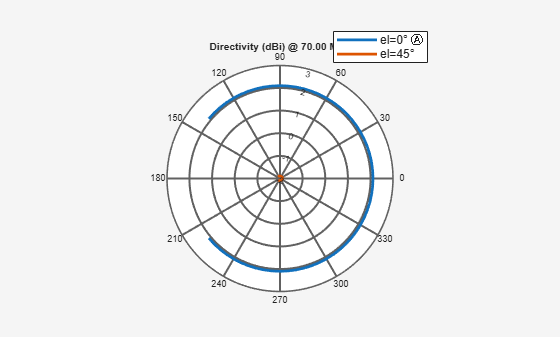# patternAzimuth

Azimuth pattern of antenna or array

## Syntax

``patternAzimuth(object,frequency,elevation)``
``patternAzimuth(object,frequency,elevation,Name,Value)``
``directivity = patternAzimuth(object,frequency,elevation)``
``directivity = patternAzimuth(object,frequency,elevation,'Azimuth')``

## Description

example

````patternAzimuth(object,frequency,elevation)` plots the 2-D radiation pattern of the antenna or array object over a specified frequency. Elevation values defaults to zero if not specified.```

example

````patternAzimuth(object,frequency,elevation,Name,Value)` uses additional options specified by one or more `Name,Value` pair arguments.```
````directivity = patternAzimuth(object,frequency,elevation)` returns the directivity of the antenna or array object over a specified frequency. Elevation values defaults to zero if not specified.```
````directivity = patternAzimuth(object,frequency,elevation,'Azimuth')` uses additional options specified by one or more `Name,Value` pair arguments.```

## Examples

collapse all

Calculate and plot the azimuth radiation pattern of the helix antenna at 2 GHz.

```h = helix; patternAzimuth(h,2e9);```Calculate and plot the azimuth radiation pattern of the dipole antenna at 70 MHz at elevation values of 0 and 45.

``` d = dipole; patternAzimuth(d,70e6,[0 45],'Azimuth',-140:5:140);```## Input Arguments

collapse all

Antenna or array object, specified as a scalar handle.

Frequency used to calculate charge distribution, specified as a scalar in Hz.

Example: 70e6

Data Types: `double`

Elevation angle values, specified as a vector in degrees.

Example: `[0 45]`

Data Types: `double`

Azimuth angles of antenna, specified as the comma-separated pair consisting of `'Azimuth'` and a vector in degrees.

Example: `'Azimuth'`,2:2:340

Data Types: `double`

## Output Arguments

collapse all

Antenna or array directivity, returned as a matrix in `dBi`. The matrix size is the product of number of elevation values and number of azimuth values.